# Ratio 11

Simplify this ratio 10 : 1/4

x =  40:1

### Step-by-step explanation: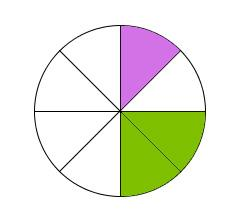Did you find an error or inaccuracy? Feel free to write us. Thank you!Tips to related online calculators
Need help to calculate sum, simplify or multiply fractions? Try our fraction calculator.
Check out our ratio calculator.

## Related math problems and questions:Why does 1 3/4 + 2 9/10 equal 4.65? How do you solve this?
• 76% is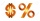76% is between which of the following two numbers. 1) 1/5 & 1/4. 2) 1/3 & 1/2 3) 2/3 & 7/10 4) 3/4 & 5/6
• Sequence 11What is the nth term of this sequence 1, 1/2, 1/3, 1/4, 1/5?
• Composite ratioJakub, Aneta, and Lenka divided 1342 USD in the ratio 5/2: 3/10: 1/4. How much did Lenka take?
• Special sequence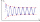What if            2×9=1            3×9=2            4×9=3            5×9=4            6×9=5            7×9=6            8×9=7            9×9=8           10×9=9    then 1×9=??? answer with solutions. .. ..
• Mrs PerryMrs Perry shares out 25 biscuits between Gemma and Zak in the ratio 1:4. How many biscuits has each?
• There 11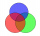There are 50 pupil in the class. Out of of this number,1/10 speak French only and 4/5 of the 10 remainder speak both French and English . If the rest speak English only find the number of students who speak only English?
• Sum three fractionsWork out the sum of 1/4, 1/5 and 3/10.
• Simplify 3Simplify mixed numerals expression: 8 1/4- 3 2/5 - (2 1/3 - 1/4) Show your solution.
• Divide moneyDivide 1200 USD at a ratio of 1:2:3:4:5:6:9:10
• The sidesThe sides of a rectangle are in a ratio of 2:3, and its perimeter is 1 1/4 inches. What are the lengths of its side? Draw it.
• Three numbers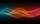The sum of the three numbers is 287. These numbers are in a ratio of 3: 7: 1/4. Find these numbers.
• Simplify 7Simplify. 7-1/3÷ 3-2/3 of 2+ 4-1/2÷ 2-1/4+ 1/2 solution and by step by stepAdd marks (+, -, *, /, brackets) to fullfill equations 1 3 6 5 = 10 This is for the 4th grade of the primary school - with no negative numbers yet(a) Convert the following mixed numbers to improper fractions. i. 3 5/8 ii. 7 7/6 (b) Convert the following improper fraction to a mixed number. i. 13/4 ii. 78/5 (c) Simplify these fractions to their lowest terms. i. 36/42 ii. 27/45 2. evaluate the follow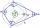What size have angles in quadrilateral (4-gon) if they are in a ratio of 8: 9: 10: 13?How many 7-digit telephone numbers can be compiled from the digits 0,1,2,..,8,9 that no digit is repeated?